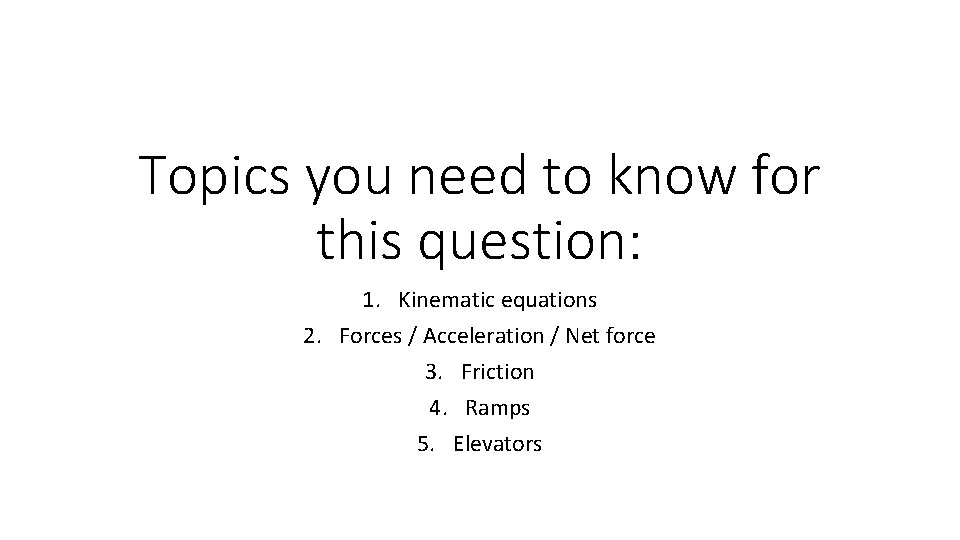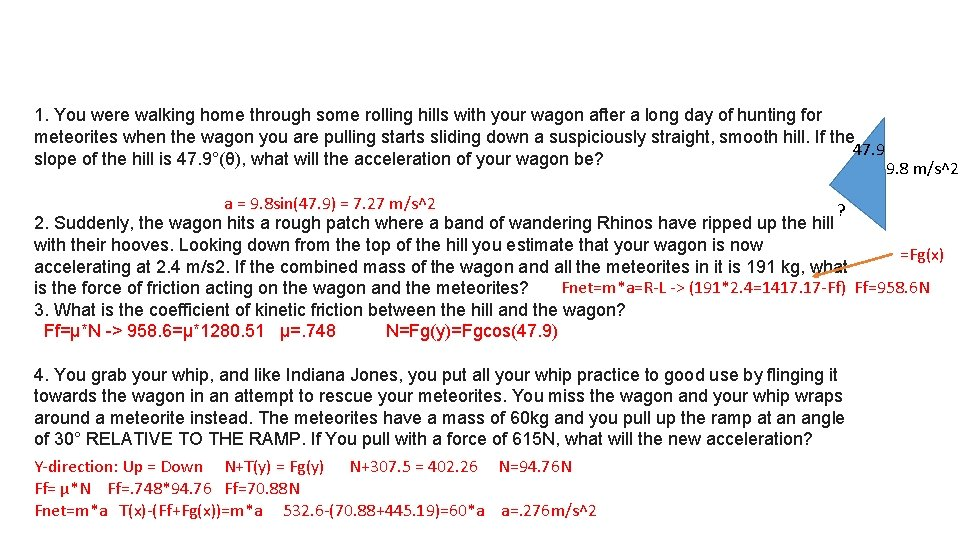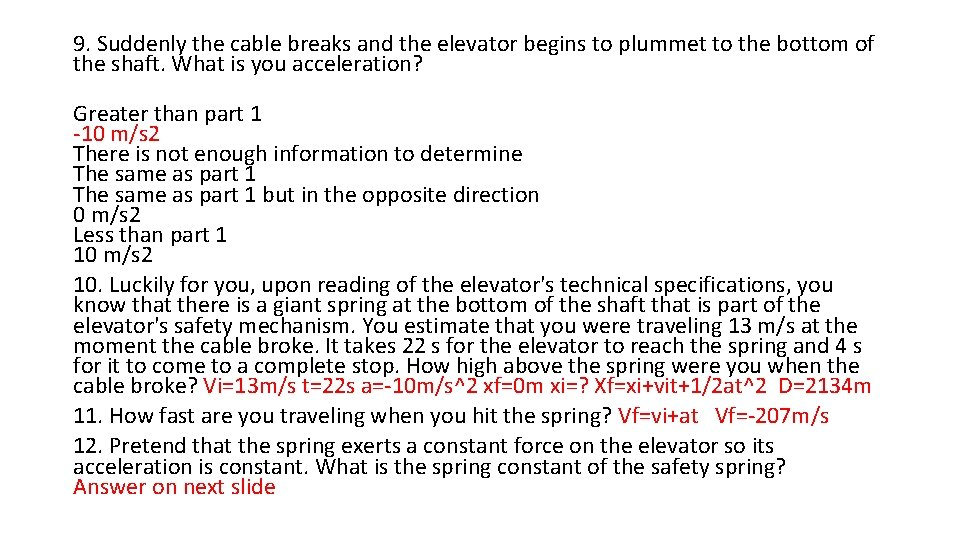# Topics you need to know for this question

• Slides: 5Topics you need to know for this question: 1. Kinematic equations 2. Forces / Acceleration / Net force 3. Friction 4. Ramps 5. Elevators1. You were walking home through some rolling hills with your wagon after a long day of hunting for meteorites when the wagon you are pulling starts sliding down a suspiciously straight, smooth hill. If the 47. 9 slope of the hill is 47. 9°(θ), what will the acceleration of your wagon be? 9. 8 m/s^2 a = 9. 8 sin(47. 9) = 7. 27 m/s^2 ? 2. Suddenly, the wagon hits a rough patch where a band of wandering Rhinos have ripped up the hill with their hooves. Looking down from the top of the hill you estimate that your wagon is now =Fg(x) accelerating at 2. 4 m/s 2. If the combined mass of the wagon and all the meteorites in it is 191 kg, what Fnet=m*a=R-L -> (191*2. 4=1417. 17 -Ff) Ff=958. 6 N is the force of friction acting on the wagon and the meteorites? 3. What is the coefficient of kinetic friction between the hill and the wagon? Ff=µ*N -> 958. 6=µ*1280. 51 µ=. 748 N=Fg(y)=Fgcos(47. 9) 4. You grab your whip, and like Indiana Jones, you put all your whip practice to good use by flinging it towards the wagon in an attempt to rescue your meteorites. You miss the wagon and your whip wraps around a meteorite instead. The meteorites have a mass of 60 kg and you pull up the ramp at an angle of 30° RELATIVE TO THE RAMP. If You pull with a force of 615 N, what will the new acceleration? Y-direction: Up = Down N+T(y) = Fg(y) N+307. 5 = 402. 26 N=94. 76 N Ff= µ*N Ff=. 748*94. 76 Ff=70. 88 N Fnet=m*a T(x)-(Ff+Fg(x))=m*a 532. 6 -(70. 88+445. 19)=60*a a=. 276 m/s^25. The wagon reaches the bottom of the hill when a door opens. The door leads into an alien’s secret underground base. Unfortunately, the door slams shut in front of you before you can run inside. Apparently the alien’s want their meteorites back. By a stroke of good fortune, you (60 kg) stubble upon a back door to the secret lair and everting the door you realize that you are in an elevator! As you step in the elevator, the door immediately closes behind you and begins moving. You look down and discovers that you are on a giant scale which reads 846 N. What is the acceleration of the elevator? Fnet=U-D (60)*a = (846)-(600) a=4. 1 m/s^2 6. What direction is the elevator moving? It's not moving. Up Right Left There is not enough information to tell. Down T-W=m*a T-3325=332. 5(4. 1) T=4688. 25 7. Etched in the door you see that this particular model of elevator, when empty, weighs 2725 N. You use that information to calculate the tension in the cable while it is accelerating. What is the tension? 8. You also notice that its maximum carrying capacity is 2093 kg and that the tensile strength of the steel cable supporting the elevator is 47226 N. What is maximum acceleration that a fully loaded elevator can have without breaking the cable? T-W=m*a 47226 -23655=2365. 5(a) a=9. 96 m/s^29. Suddenly the cable breaks and the elevator begins to plummet to the bottom of the shaft. What is you acceleration? Greater than part 1 -10 m/s 2 There is not enough information to determine The same as part 1 but in the opposite direction 0 m/s 2 Less than part 1 10 m/s 2 10. Luckily for you, upon reading of the elevator's technical specifications, you know that there is a giant spring at the bottom of the shaft that is part of the elevator's safety mechanism. You estimate that you were traveling 13 m/s at the moment the cable broke. It takes 22 s for the elevator to reach the spring and 4 s for it to come to a complete stop. How high above the spring were you when the cable broke? Vi=13 m/s t=22 s a=-10 m/s^2 xf=0 m xi=? Xf=xi+vit+1/2 at^2 D=2134 m 11. How fast are you traveling when you hit the spring? Vf=vi+at Vf=-207 m/s 12. Pretend that the spring exerts a constant force on the elevator so its acceleration is constant. What is the spring constant of the safety spring? Answer on next slide-> t=4 s Vf=0 Vi=-207 Xf=? Xi=o ΔX=-414 m -> xf=xi+1/2(Vi+Vf)t Xf=414 m -> Ke=1/2 mv^2 Ug=mgh Us=1/2 kx^2 Ke+Ug=Us ½(332. 5)(207)^2+(332. 5)(10)(414)=1/2 k(414)^2 K=99. 19